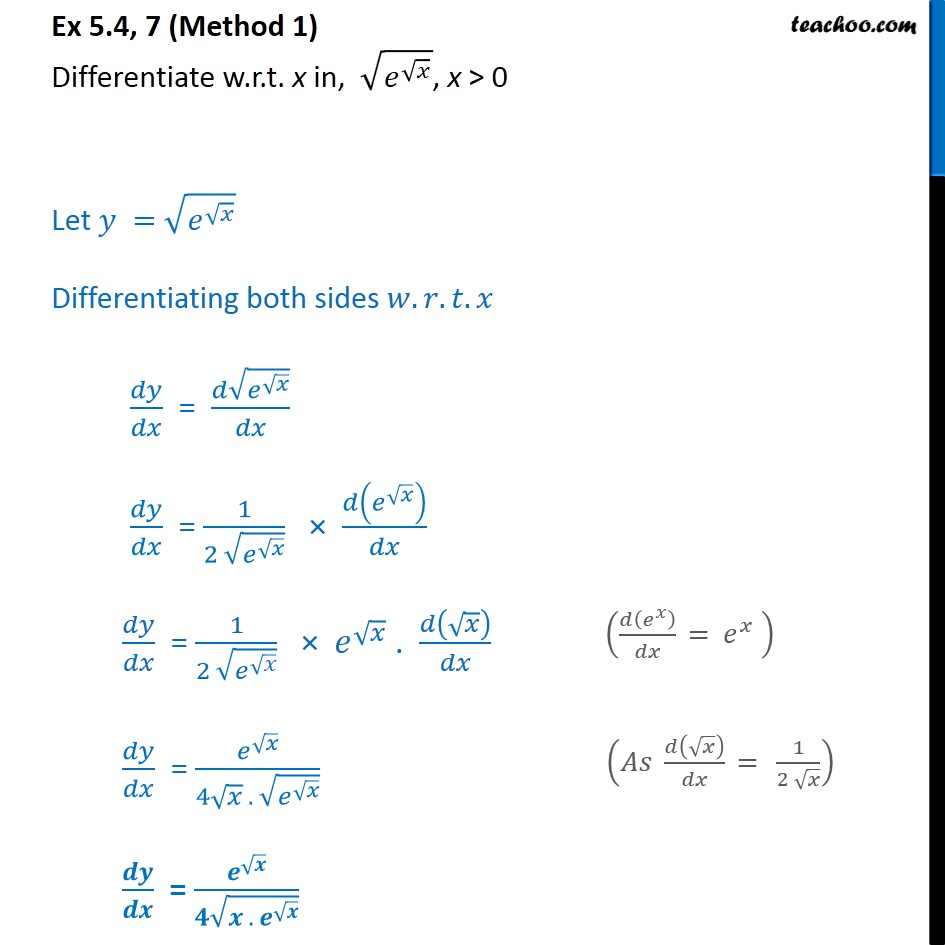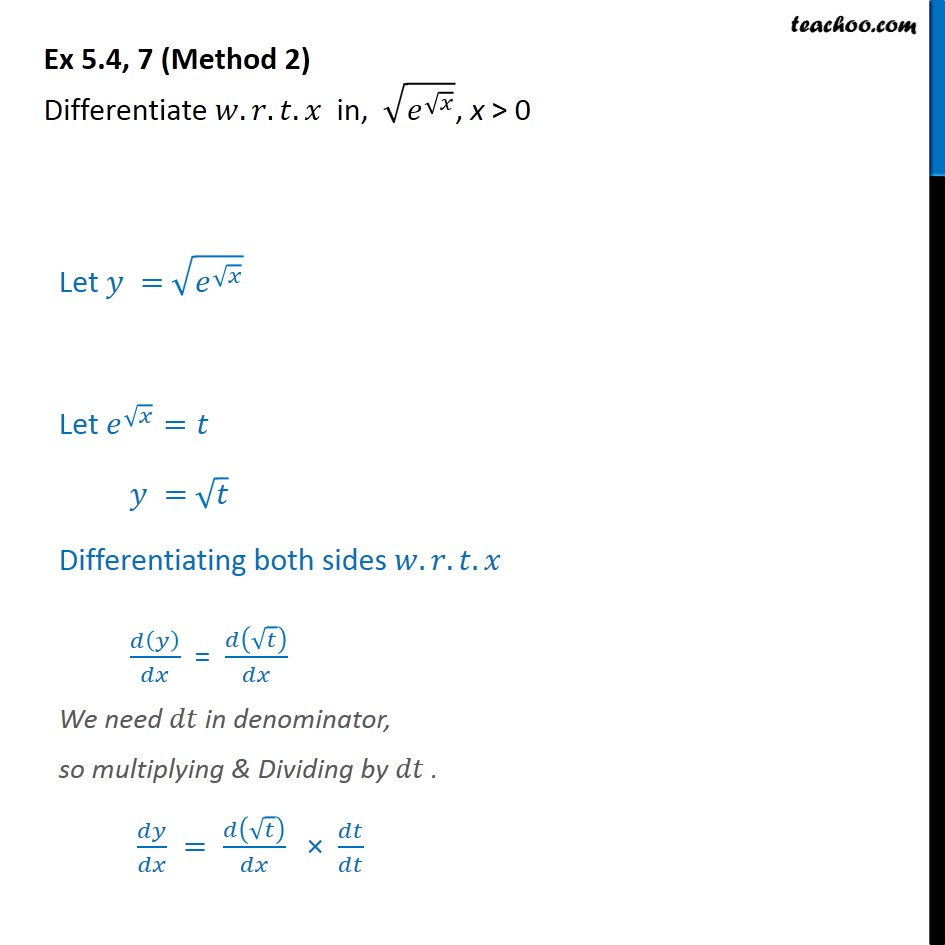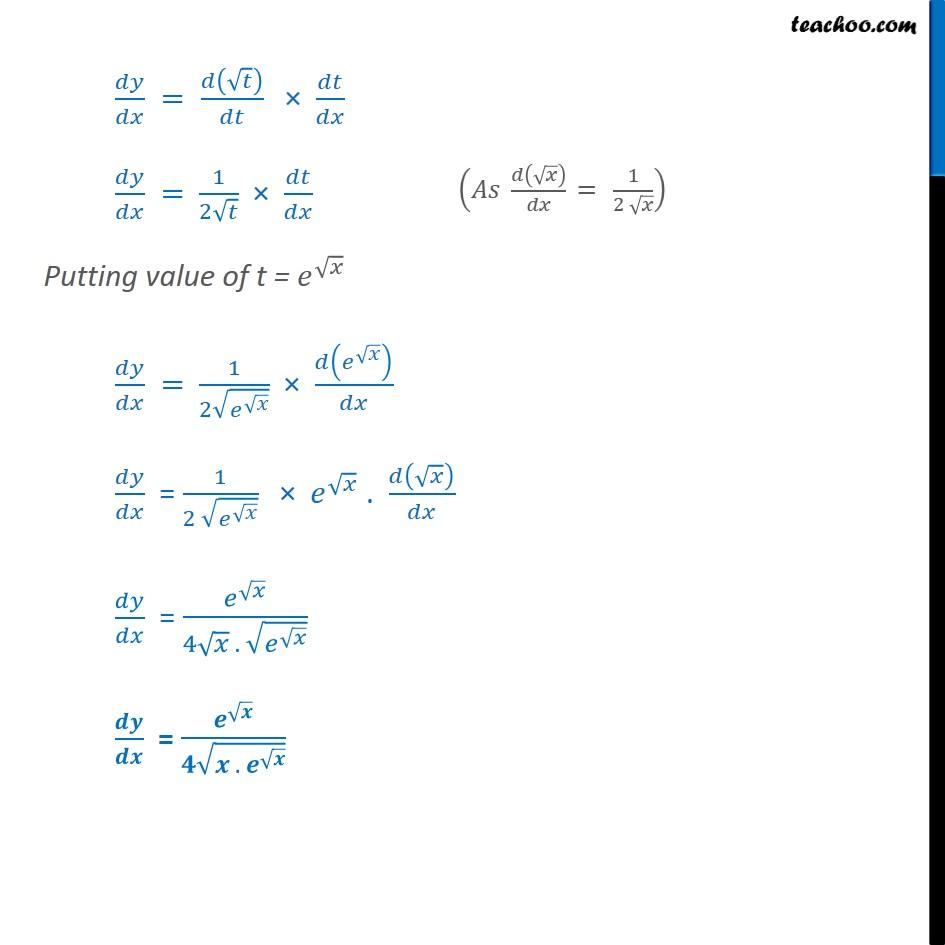1. Chapter 5 Class 12 Continuity and Differentiability
2. Serial order wise
3. Ex 5.4

Transcript

Ex 5.4, 7 (Method 1) Differentiate w.r.t. x in, ﷮ 𝑒﷮ ﷮𝑥﷯﷯﷯, x > 0 Let 𝑦 = ﷮ 𝑒﷮ ﷮𝑥﷯﷯﷯ Differentiating both sides 𝑤.𝑟.𝑡.𝑥 𝑑𝑦﷮𝑑𝑥﷯ = 𝑑 ﷮ 𝑒﷮ ﷮𝑥﷯﷯﷯﷮𝑑𝑥﷯ 𝑑𝑦﷮𝑑𝑥﷯ = 1﷮2 ﷮ 𝑒﷮ ﷮𝑥﷯﷯﷯﷯ × 𝑑 𝑒﷮ ﷮𝑥﷯﷯﷯﷮𝑑𝑥﷯ 𝑑𝑦﷮𝑑𝑥﷯ = 1﷮2 ﷮ 𝑒﷮ ﷮𝑥﷯﷯﷯﷯ × 𝑒﷮ ﷮𝑥﷯﷯ . 𝑑 ﷮𝑥﷯﷯﷮𝑑𝑥﷯ 𝑑𝑦﷮𝑑𝑥﷯ = 𝑒﷮ ﷮𝑥﷯﷯﷮4 ﷮𝑥﷯ . ﷮ 𝑒﷮ ﷮𝑥﷯﷯﷯﷯ 𝒅𝒚﷮𝒅𝒙﷯ = 𝒆﷮ ﷮𝒙﷯﷯﷮𝟒 ﷮𝒙 . 𝒆﷮ ﷮𝒙﷯﷯﷯﷯ Ex 5.4, 7 (Method 2) Differentiate 𝑤.𝑟.𝑡. 𝑥 in, ﷮ 𝑒﷮ ﷮𝑥﷯﷯﷯, x > 0 Let 𝑦 = ﷮ 𝑒﷮ ﷮𝑥﷯﷯﷯ Let 𝑒﷮ ﷮𝑥﷯﷯=𝑡 𝑦 = ﷮𝑡﷯ Differentiating both sides 𝑤.𝑟.𝑡.𝑥 𝑑 𝑦﷯﷮𝑑𝑥﷯ = 𝑑 ﷮𝑡﷯﷯﷮𝑑𝑥﷯ We need 𝑑𝑡 in denominator, so multiplying & Dividing by 𝑑𝑡 . 𝑑𝑦﷮𝑑𝑥﷯ = 𝑑 ﷮𝑡﷯﷯﷮𝑑𝑥﷯ × 𝑑𝑡﷮𝑑𝑡﷯ 𝑑𝑦﷮𝑑𝑥﷯ = 𝑑 ﷮𝑡﷯﷯﷮𝑑𝑡﷯ × 𝑑𝑡﷮𝑑𝑥﷯ 𝑑𝑦﷮𝑑𝑥﷯ = 1﷮2 ﷮𝑡﷯﷯ × 𝑑𝑡﷮𝑑𝑥﷯ Putting value of t = 𝑒﷮ ﷮𝑥﷯﷯ 𝑑𝑦﷮𝑑𝑥﷯ = 1﷮2 ﷮ 𝑒﷮ ﷮𝑥﷯﷯﷯﷯ × 𝑑 𝑒﷮ ﷮𝑥﷯﷯﷯﷮𝑑𝑥﷯ 𝑑𝑦﷮𝑑𝑥﷯ = 1﷮2 ﷮ 𝑒﷮ ﷮𝑥﷯﷯﷯﷯ × 𝑒﷮ ﷮𝑥﷯﷯ . 𝑑 ﷮𝑥﷯﷯﷮𝑑𝑥﷯ 𝑑𝑦﷮𝑑𝑥﷯ = 𝑒﷮ ﷮𝑥﷯﷯﷮4 ﷮𝑥﷯ . ﷮ 𝑒﷮ ﷮𝑥﷯﷯﷯﷯ 𝒅𝒚﷮𝒅𝒙﷯ = 𝒆﷮ ﷮𝒙﷯﷯﷮𝟒 ﷮𝒙 . 𝒆﷮ ﷮𝒙﷯﷯﷯﷯

Ex 5.4